# Difference: SupplyChainMP (11 vs. 12)

#### Revision 122008-04-01 - MichaelOSullivan

Line: 1 to 1

 META TOPICPARENT name="SupplyChains"
>
>
<-- Ready to Review -->
%BEGINLATEXPREAMBLE% \usepackage{amsmath} \usepackage{amssymb}
Line: 115 to 117
If the prices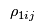,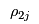and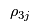are not coordinated then solving the Manufacturer's Problem, the Retailer's Problem and the Consumers' Problem will give inconsistent values for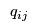and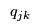. That is, the manufacturers will want to ship different ammounts to the retailers than the retailers will want to receive from the maunfacturers and the retailers will want to sell different amounts to the demand markets from the amounts that the consumers at the demand markets want to purchase. However, these prices will adjust until the supply chain reaches equilibrium and the flows from the different mathematical programmes agree.
Changed:
<
<
One way to find this equilibrium point is to solve a [[VariationalInequalities][variational inequality]. Theorem 1 gives the necessary variational inequalities:
>
>
One way to find this equilibrium point is to solve a [[https://en.wikipedia.org/wiki/Variational_inequality][variational inequality]. Theorem 1 gives the necessary variational inequalities:
Theorem 1: Variational Inequality The equilibrium state governing the supply chain model according to the optimality conditions of the supply chain mathematical programmes (?? REFLATEX{eq:manufacturer} not defined in eqn list ??), (?? REFLATEX{eq:retailer} not defined in eqn list ??) and (?? REFLATEX{eq:consumer} not defined in eqn list ??) is equivalent to the solution of the variational inequality problem given by: determine the equilibrium vectors of product shipments, shadow prices, and demand market prices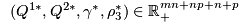satisfying
>
>
%BEGINLATEX% \begin{equation*} \begin{split}
Line: 129 to 132
\end{equation*} %ENDLATEX%
>
>
Once the equilibrium state has been found the manufacturers' prices and the retailers' prices can be calculated:
•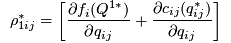if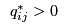;
•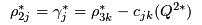if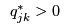for some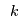.